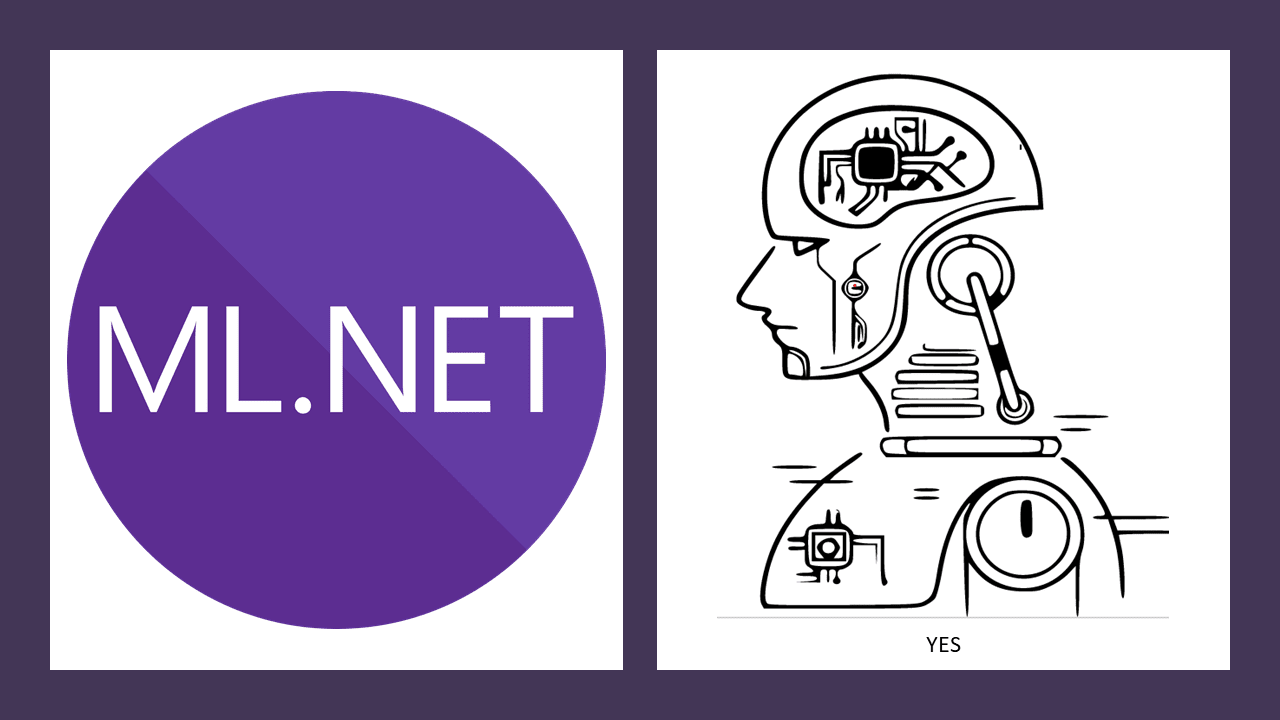# ML.NET and Python Polynomial Linear RegressionThis is the fourth in a series talking about differences implementing machine learning tasks in ML.NET and Python

Here are the previous articles if you'd like to peruse them

## What is Polynomial Linear Regression?

Polynomial linear regression is a bit of a strange customer. Where before our regressions could be consistently described as lines, a polynomial regression is a curve. A polynomial regression is appropriate when there is exponential growth in the dependent variable.

So something like this simple example:

IndependentDependent
14
28
316
432
564

Our data looks the same as simple linear regression at first, but upon noticing exponential growth in the dependent variable we need a different equation. A linear regression line will not be accurate. In fact, observing a regression line from a typical simple regression may be how we can identify the need for a polynomial regression.

On data such as this we would need to determine the correct exponent for growth and then apply it to the independent variable.

In order to perform the regression we need to expand out the independent variable by expanding this equation out for each possible result of 0 - {possible exponent value}. In practice such a transformation would change the above example into something like this.

Independent1Independent2Independent3Dependent
1114
1248
13916
141632
152564

The reason we still call this linear regression despite the fact the growth curve is exponential is because the independent variables are still expressed linearly. Basically when we see `1, 2, 3, 4 ...` and can say

"Ok, this variable is growing in a linear way."

Then, even though our dependent variable is growing exponentially we still consider this a linear regression.

Polynomial regression is commonly used for tasks such as predicting disease spread in a population.